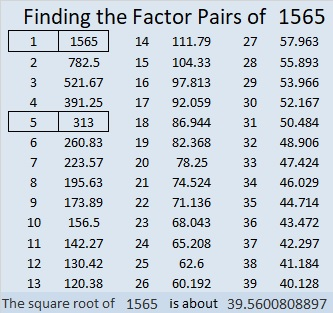# 1565 Stable with Manger

### Today’s Puzzle:

This mystery level puzzle reminds me of the manger in the stable that first Christmas night.

How difficult will the puzzle be to solve? That is part of the mystery. You will have to try it for yourself to find out.### Factors of 1565:

• 1565 is a composite number.
• Prime factorization: 1565 = 5 × 313.
• 1565 has no exponents greater than 1 in its prime factorization, so √1565 cannot be simplified.
• The exponents in the prime factorization are 1 and 1. Adding one to each exponent and multiplying we get (1 + 1)(1 + 1) = 2 × 2 = 4. Therefore 1565 has exactly 4 factors.
• The factors of 1565 are outlined with their factor pair partners in the graphic below.### More about the Number 1565:

1565 is the sum of two squares in two different ways:
37² + 14² = 1565, and
38² + 11² = 1565.

125-1560-1565, which is 5 times (25-312-313),
836-1323-1565, calculated from 2(38)(11), 38² – 11², 38² + 11²,
939-1252-1565, which is (3-4-5) times 313, and
1036-1173-1565, calculated from 2(37)(14), 37² – 14², 37² + 14².